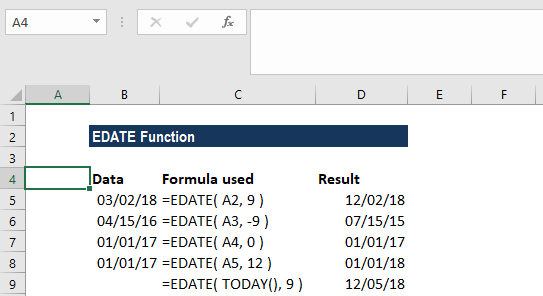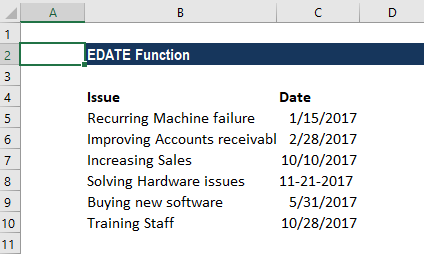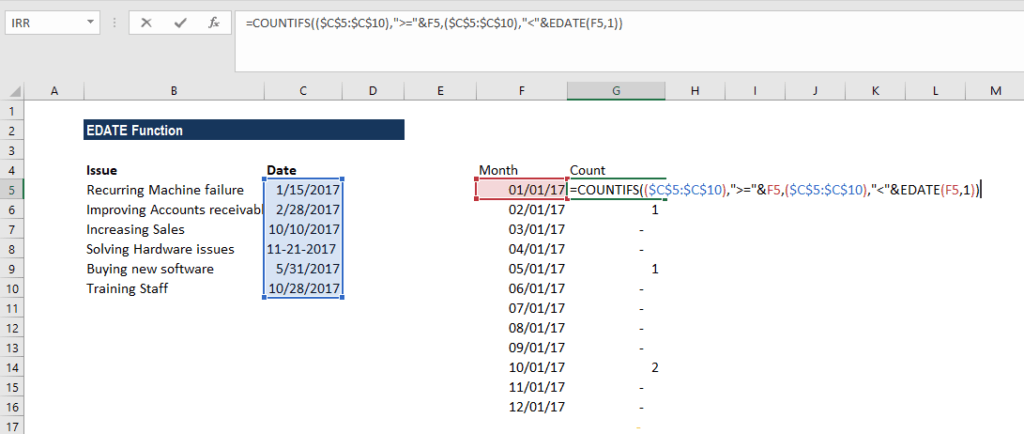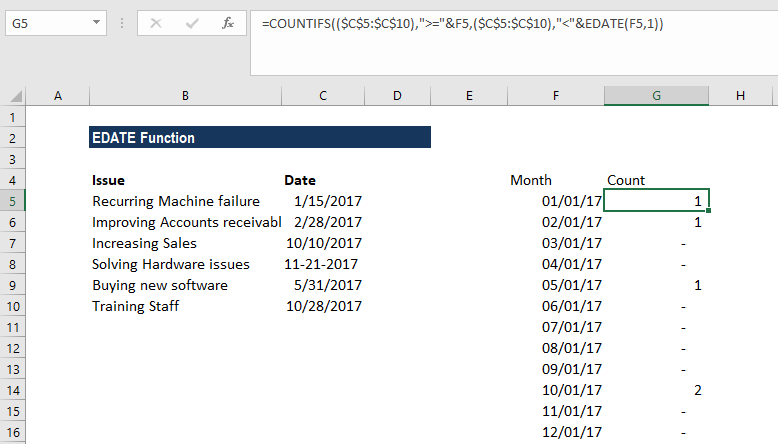# EDATE Function

Returns a serial date from adding a specified number of months to a date

## What is the EDATE Function?

The EDATE Function is categorized under Excel DATE/TIME Functions. The function helps add a specified number of months to a date and returns the result as a serial date. Apart from helping a financial analyst calculate maturity dates for accounts payable or accounts receivable that fall on the last day of the month, the EDATE function also helps in calculating summary count by month.

### Formula

=EDATE(start_date, months)

The EDATE function includes the following arguments:

1. Start_date (required argument) – This is the initial date. We need to enter dates in date format, either by using the DATE function or as results of other formulas or functions. For example, use DATE(2017,5,24) for May 24, 2017. The function will return errors if dates are entered as text., as Excel interprets text representations of dates differently, depending on the date interpretation settings on the computer.
2. Months (required argument) – This is the number of months before or after the start_date. A positive value for months yields a future date; a negative value produces a past date.

### How to use the EDATE Function in Excel?

To understand the uses of this function, let’s consider a few examples:

#### Example 1

Let’s see what results we get when we use the following data:In Row 2, the function returned a date that is 9 months after the start date. In Row 3 it returned a day that is 9 months before the start date.

In row 4 as we were given months as zero, so it returned the date the same as the start date. Whereas in row 6, we used Today function along with EDATE function. So, it returned a date that is 8 months from today.

Remember that the EDATE function will return a serial date value. A serial date is how Excel stores dates internally and it represents the number of days since January 1, 1900.

#### Example 2 – Using EDATE with other functions

Assume we are a start-up company with a list of issues and due dates by which we would solve them. If we wish to create a summary count by month, we can use COUNTIF and EDATE.

The data given are as follows:As seen above, we assigned due dates to tasks/issues listed above. To create a summary by month, we can use the formula involving COUNTIFS and EDATE.

The formula to use is =COUNTIFS((\$B\$3:\$B\$8),”>=”&E3,(\$B\$3:\$B\$8),”<“&EDATE(E3,1)).The result we would get would be the number of tasks to be done monthly for the entire year, as shown below:In the example above, we see a list of six tasks in Column A, each with a corresponding target date.

From column E, we get a summary table that shows a total count per month. The first column of the summary table is a date for the first of each month in 2017. In formulas, for COUNTIFS, we need to supply the criteria that capture all data by date.  To generate a total count per month, we need the criteria that counts all tasks to be completed in an entire year by month.

Using the actual dates in Column E, we can construct the criteria using the date itself. The second criteria will be created by the EDATE function. These two criteria appear inside COUNTIFS like:

dates,”>=”&E5,(B3:B8),”<“&EDATE(E5,1)

The formula above is roughly translated as “dates greater than or equal to the date in E5 and less than the date in E5 plus one month.” It is a convenient way to generate “brackets” for each month with one date only. COUNTIFS generates the correct count for each month.

Remember that if we do not wish to see full dates in column E, we can apply the custom date formats “mmm” or “mmmm” to display month names only.

### Things to remember about the EDATE Function

1. #NUM! error – Occurs when the date resulting from the calculation is not a valid date.
2. #VALUE error – Occurs if:
1. The supplied start_date is not a valid Excel date; or
2. Any of the supplied arguments are non-numeric.
3. If we provide a decimal value for months, the EOMONTH function will only add the integer portion to start_date.

Thanks for reading CFI’s guide to important Excel functions! By taking the time to learn and master these functions, you’ll significantly speed up your financial analysis. To learn more, check out these additional CFI resources:

• Excel Functions for Finance